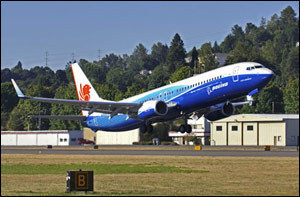# Boeing 737 Dimensions

The size of the Boeing 737 and its specifications differ per model. The proceeding information is for the specified models; they do not necessarily apply to other 737s.

### Dimensions of the Boeing 737 900

The 737 900 has a seatingcapacity of 189 (1 class, dense) and 177 (1 class standard). The length is 138 ft 2 in (42.1 m) with a wingspan of 117 ft 5 in (35.7 m). The 737 900’s tail height is 41 ft 2 in (2.5 m).

Its wing sweepback is 25.02 degrees. Its fuselage width is 12 ft 4 in (equal to 3.8 m) and the fuselage height is 13 ft 2 in (4.0 m). The cabin width is 11 ft 7 in (equivalent to 3.5 m).

The size of the Boeing 737 900 gives it an empty weight of 94,580 lbs (42,901 kg). The maximum take off weight is about 174,200 lbs or 79,016 kg. The maximum loading weight is 146,300 lbs (6,361 kg).

The 737 900’s cargo capacity is 1,852 sq ft (52.5 m3). The cruising speed is Mach 0.785 (828 km/h). The maximum fuel capacity is 6,875 US gallons (26,020 l).

### Dimensions of the Boeing 737 300

The 737 300 has a capacity of 149 (1 class, dense) and 128 (1 class, standard). Its length is 109 ft 7 in (33.4 m). The seat pitch is 17.2” with a wingspan of 94 ft 8 in (28.88 m). The size of the Boeing 737 gives it a height of 36 ft 6 in (11.13 m).

Its fuselage width is 12 ft 4 in (3.76 m) and the height is 13 ft 6 in (4.11 m). The cabin width is 11 ft 7 in (3.54 m). The cabin’s height is 7 ft 3 in (2.20 m).

Its empty weight is 72,100 lbs (32,700 kg) and the take off weight
is 138,500 lbs or 62,820 kg. The maximum landing weight of the 737 300 is 114,000 lbs or 51,700 kg. Its cargo capacity is 82 sq ft (equal to 23.3 m3).

The 737 300’s cruise speed is Mach 0.745 (maximum Mach speed is 0.82). The fuel capacity is 6,130 US gallons (23,170 l). Its engine length is 93 in (2.36 m).

### Dimensions of the Boeing 737 600

The size of the Boeing 737 600 (one of the Boeing 737 Next Generation series) allows for a max take off weight of 145,000 lbs (66,000 kg). The length is 102 ft 6 in (31.2 m) with a wingspan of 117 ft 5 in (35.7 m). The height is 41 ft 3 in (or 12.6 m). The wing sweepback is 25.02 degrees with an aspect ratio of 9.45.

The fuselage height is 13 ft 2 in (4.01 m). The fuselage width is 12 ft 4 in (3.76 m). The cabin height and width is 7 ft 3 in (2.20 m) and 11 ft 7 in (3.54 m) respectively. Its maximum speed is Mach 0.82 (878 km/h, 544 mphor 473 knots). The cruising speed is 828 km/h.

The size of the Boeing 737 as given here may change, as the manufacturer may undertake modifications to the models.